Test: The Triangle and Its Properties

# Test: The Triangle and Its Properties

Test Description

## 15 Questions MCQ Test Mathematics (Maths) Class 7 | Test: The Triangle and Its Properties

Test: The Triangle and Its Properties for Class 7 2023 is part of Mathematics (Maths) Class 7 preparation. The Test: The Triangle and Its Properties questions and answers have been prepared according to the Class 7 exam syllabus.The Test: The Triangle and Its Properties MCQs are made for Class 7 2023 Exam. Find important definitions, questions, notes, meanings, examples, exercises, MCQs and online tests for Test: The Triangle and Its Properties below.
Solutions of Test: The Triangle and Its Properties questions in English are available as part of our Mathematics (Maths) Class 7 for Class 7 & Test: The Triangle and Its Properties solutions in Hindi for Mathematics (Maths) Class 7 course. Download more important topics, notes, lectures and mock test series for Class 7 Exam by signing up for free. Attempt Test: The Triangle and Its Properties | 15 questions in 10 minutes | Mock test for Class 7 preparation | Free important questions MCQ to study Mathematics (Maths) Class 7 for Class 7 Exam | Download free PDF with solutions
 1 Crore+ students have signed up on EduRev. Have you?
Test: The Triangle and Its Properties - Question 1

### How many medians a triangle can have?

Detailed Solution for Test: The Triangle and Its Properties - Question 1

In geometry, a median of a triangle is a line segment joining a vertex to the midpoint of the opposite side, thus bisecting that side. Every triangle has exactly three medians, one from each vertex, and they all intersect each other at the triangle's centroid.

Test: The Triangle and Its Properties - Question 2

### A/an _____________ connect a vertex of a triangle to the mid-point of the opposite side.

Detailed Solution for Test: The Triangle and Its Properties - Question 2

A/an median  connect a vertex of a triangle to the mid-point of the opposite side.

Test: The Triangle and Its Properties - Question 3

### How many altitudes can a triangle have?

Detailed Solution for Test: The Triangle and Its Properties - Question 3

A triangle can have 3 altitudes

Test: The Triangle and Its Properties - Question 4

ΔABC is right-angled at C. If AC = 5 cm and BC = 12 cm find the length of AB.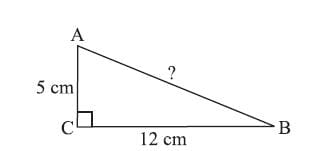Detailed Solution for Test: The Triangle and Its Properties - Question 4

By Pythagoras property,
AB2 = AC2 + BC2
= 52 + 122 = 25 + 144 = 169 = 132
or AB2= 132. So, AB = 13 or the length of AB is 13 cm.

Test: The Triangle and Its Properties - Question 5

Side opposite to the vertex Q of ΔPQR is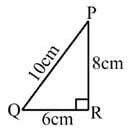Detailed Solution for Test: The Triangle and Its Properties - Question 5

In triangle PQR, for vertex Q the line joining the other two vertex is the side opposite to Q.

for example,Test: The Triangle and Its Properties - Question 6

Vertex opposite to the side RT of ΔRST is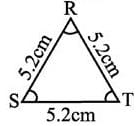Detailed Solution for Test: The Triangle and Its Properties - Question 6

The vertex opposite to side RT is S.

Step-by-step explanation:

In the given question,

We have a triangle RST which has sides RS, ST and TR.

We know that a triangle consists three vertices and three sides each of which is particularly opposite to any one of the side of the triangle and vice-versa.

Therefore, we can see here that in the triangle RST.

The side RS is opposite to the vertex T.

The side ST is opposite to the vertex R.

and,

The side TR is opposite to the vertex S.

Hence, the correct answer will be S as side RT is opposite to S.

Test: The Triangle and Its Properties - Question 7

In Δ PQR, PM is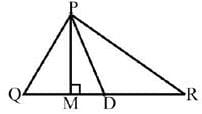Detailed Solution for Test: The Triangle and Its Properties - Question 7

In Δ PQR, PM is altitude

Test: The Triangle and Its Properties - Question 8

Find the value of x in the adjoining figure.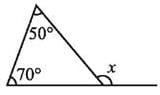Detailed Solution for Test: The Triangle and Its Properties - Question 8

An exterior angle of a triangle is equal to the sum of the opposite interior angles

xo = 70o + 50o

xo = 120o .

So option C is the correct answer.

Test: The Triangle and Its Properties - Question 9

Find the value of x.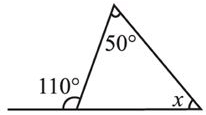Detailed Solution for Test: The Triangle and Its Properties - Question 9

An exterior angle of a triangle is equal to the sum of the opposite interior angles

So in the given figure

110o = 50o + x

110o - 50o = x

60o = x

So option B is correct answer.

Test: The Triangle and Its Properties - Question 10

Find the value of x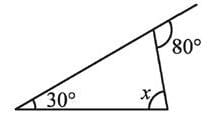Detailed Solution for Test: The Triangle and Its Properties - Question 10

An exterior angle of a triangle is equal to the sum of the opposite interior angles

So in the given figure

80o = 30o + x

it implies x = 50o

Test: The Triangle and Its Properties - Question 11

If the angles of a triangle are in the ratio 4:5:9. Find all the angles of a the triangle

Detailed Solution for Test: The Triangle and Its Properties - Question 11

The sum of the angles of a triangle is 180 degrees. As the ratio of the angles of the triangle is 4:5:9 they can be taken to be 4x, 5x and 9x. 4x + 5x + 9x = 180

=> 18x = 180

=> x = 10

This gives the angles of the triangle as 4*10=40,

5*10=50 and 9*10=90 degrees.

Test: The Triangle and Its Properties - Question 12

The triangle whose lengths of sides are 3 cm, 4 cm, 5 cm is a

Detailed Solution for Test: The Triangle and Its Properties - Question 12

a2+b2=c2

we find 32+42=52

9 + 16 = 25

So, these sides also cause it to be a right triangle.

Test: The Triangle and Its Properties - Question 13

One of the angles of a triangle is 110° and the other two angles are equal what is the measure of each of these equal angles

Detailed Solution for Test: The Triangle and Its Properties - Question 13

One angle of the triangle = 110°
Let the other two angles each be x
Sum of all the angles of a triangle = 180°
x + x + 110 = 180
2x = 180 - 110
2x = 70
x = 35°
The other two angles are 35°, 35°.

Test: The Triangle and Its Properties - Question 14

The measure of three angles of a triangle are in the ratio 5:3:1.find the measure of this angles

Detailed Solution for Test: The Triangle and Its Properties - Question 14

Let the angles be x
sum of the angles of a triangle=180

5x+3x+x=180
9x=180
x=20
angle I = 5x = 5*20 = 100
angle II = 3x = 3*20 = 60
angle III = x = 20

Test: The Triangle and Its Properties - Question 15

The altitude and median be same for a which triangle?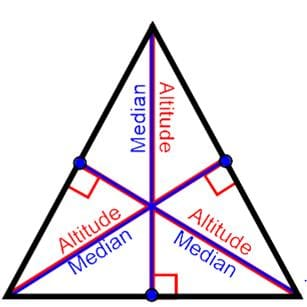Detailed Solution for Test: The Triangle and Its Properties - Question 15

Since the sides of the isosceles triangle are equal the line drawn from vertex to middle of the opposite side which is median is perpendicular. And altitude is also perpendicular . So both are the same in the isosceles triangle.

## Mathematics (Maths) Class 7

168 videos|276 docs|45 tests
 Use Code STAYHOME200 and get INR 200 additional OFF Use Coupon Code
Information about Test: The Triangle and Its Properties Page
In this test you can find the Exam questions for Test: The Triangle and Its Properties solved & explained in the simplest way possible. Besides giving Questions and answers for Test: The Triangle and Its Properties, EduRev gives you an ample number of Online tests for practice

## Mathematics (Maths) Class 7

168 videos|276 docs|45 tests